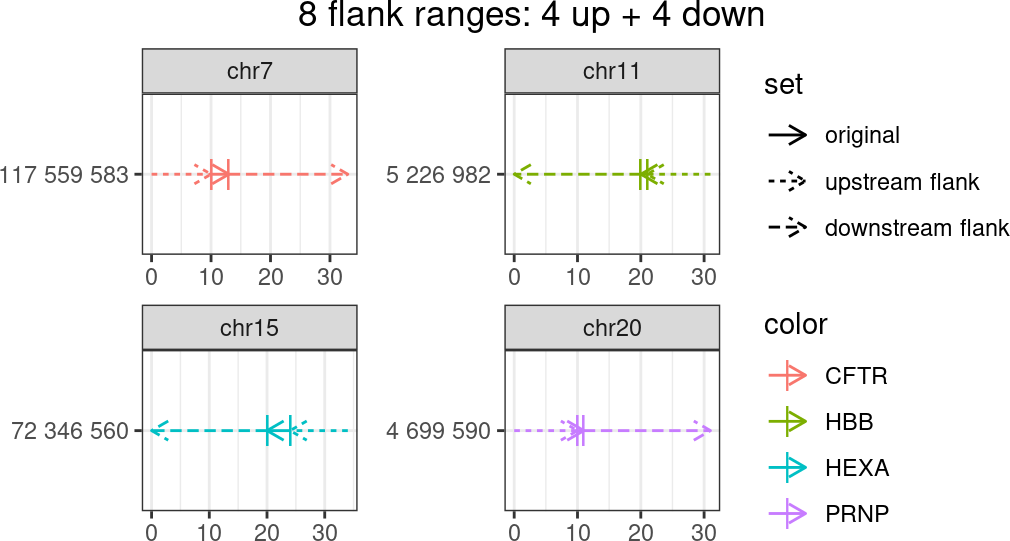# Introduction

This vignette discusses multicrispr’s genome arithmetics functionality, often required in the first step of Crispr/Cas9 design. Multicrispr’s genome arithmetics functions allow two-way transformations around a genomic range’s start/end. The GenomicRanges and plyranges packages also offer an extensive genome arithmetics functionality, though with functions designed as one-way transformations.

We illustrate the different operations on a small GRanges with four prime editing targets in Anazlone et al. (2019)

require(multicrispr)
bsgenome <- BSgenome.Hsapiens.UCSC.hg38::BSgenome.Hsapiens.UCSC.hg38
gr <- char_to_granges(c(PRNP  = 'chr20:4699600:+',         # snp
HBB  = 'chr11:5227002:-',            # snp
HEXA = 'chr15:72346580-72346583:-',  # del
CFTR = 'chr7:117559593-117559595:+'),# ins
bsgenome = bsgenome)
plot_intervals(gr, facet_var = c('targetname','seqnames'))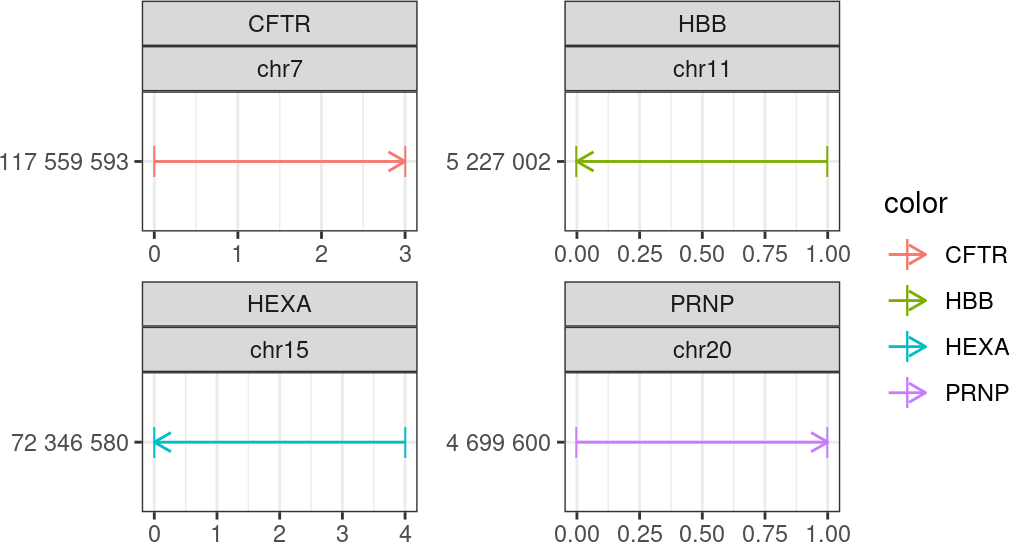# Genome arithmetics

## Extend

ext <- extend(gr, start = -10, end = +10, plot = TRUE)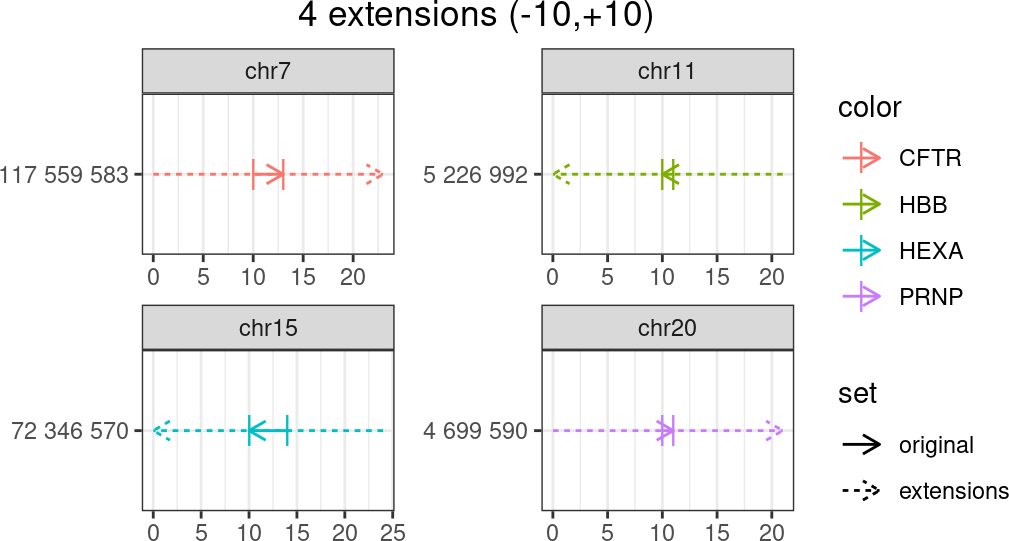## Up flank

up <- up_flank(gr,  -22,  -1, plot=TRUE, facet_var=c('targetname', 'seqnames'))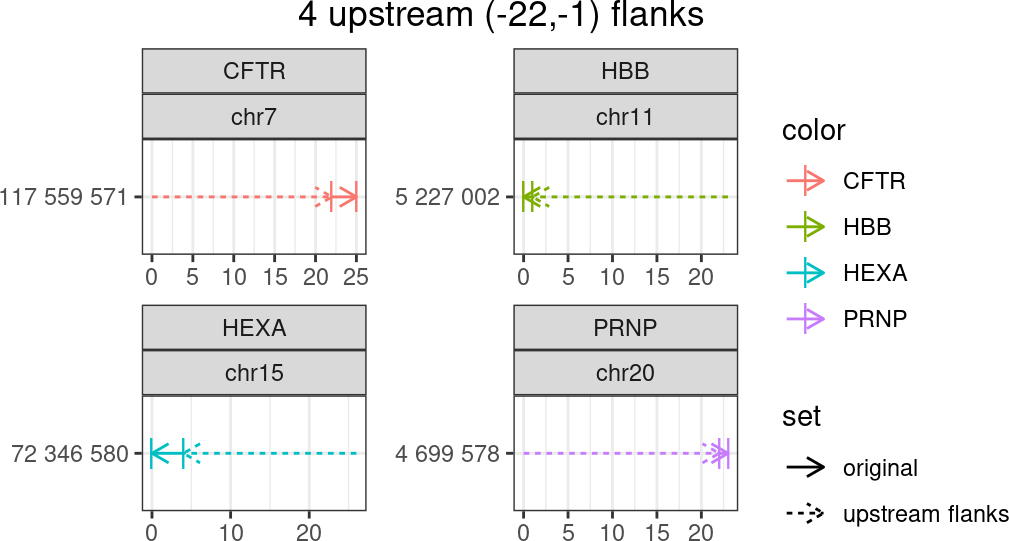## Down flank

dn <- down_flank(gr, +1, +22, plot=TRUE)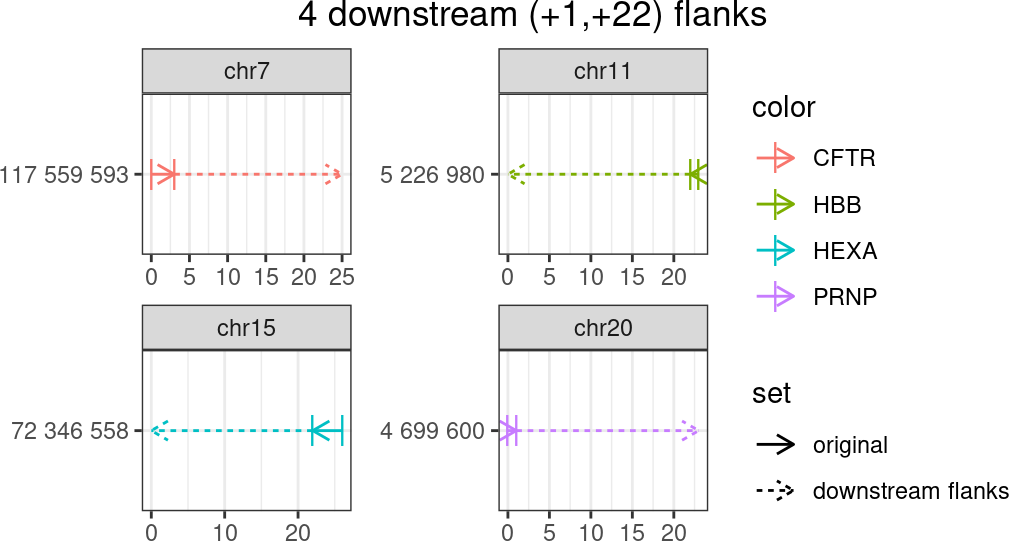## Double flank

dbl <- double_flank(gr, -10,  -1, +1, +20, plot = TRUE)
##      8 flank ranges: 4 up + 4 down